Courses

# Integer Answer Type Questions: Organic Chemistry - Some Basic Principles & Technique | JEE Advanced Notes | EduRev

## JEE : Integer Answer Type Questions: Organic Chemistry - Some Basic Principles & Technique | JEE Advanced Notes | EduRev

The document Integer Answer Type Questions: Organic Chemistry - Some Basic Principles & Technique | JEE Advanced Notes | EduRev is a part of the JEE Course Class 11 Chemistry 35 Years JEE Mains &Advance Past yr Paper.
All you need of JEE at this link: JEE

Q.1. The total number of cyclic structural as well as stereo isomers possible for a compound with the molecular formula C5H10 is

Ans. 7

Solution. The seven possible cyclic structural and stereoisomers are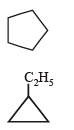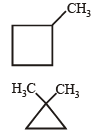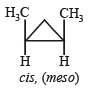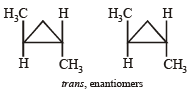Q. 2. The total number of cyclic isomers possible for a hydrocarbon with the molecular formula C4H6 is 5.

Ans. 5

Solution. The number of cyclic isomers for a hydr ocarbon with molecular formula C4H6 is 5.

The structures are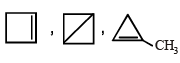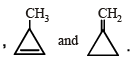Q. 3. The maximum number of isomers (including stereoisomers) that are possible on monochlorination of the following compound is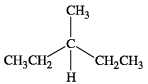Ans. 8

Solution.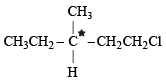Enantiomeric pair = 2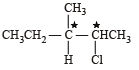Two enantiomeric pairs = 4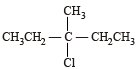= 1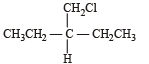= 1

Total = 2 + 4 + 1 + 1 = 8

Q. 4. The total number of contributing structures showing hyperconjugation (involving C-H bonds) for the following carbocation is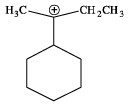Ans. 6

Solution.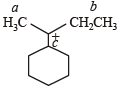a = 3 Hyperconjugative H's
b = 2 Hyperconjugative H's
c = 1 Hyperconjugative H

Q. 5. The total number(s) of stable conformers with non-zero dipole moment for the following compound is (are)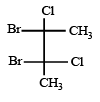Ans. 3

Solution.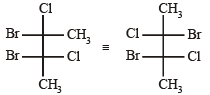Following three conformers (with μ ≠ 0) are possible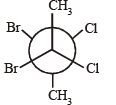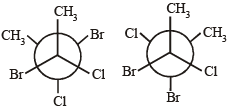Q. 6. The total number of stereoisomers that can exist for M is

Ans. 2

Solution. The molecule cannot show geometrical isomerism, so only its mirror image will be the other stereoisomer.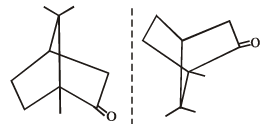Offer running on EduRev: Apply code STAYHOME200 to get INR 200 off on our premium plan EduRev Infinity!

## Class 11 Chemistry 35 Years JEE Mains &Advance Past yr Paper

122 docs|66 tests

,

,

,

,

,

,

,

,

,

,

,

,

,

,

,

,

,

,

,

,

,

;